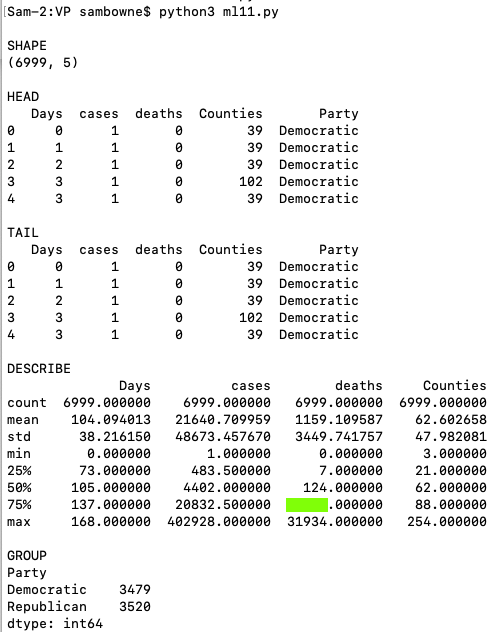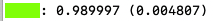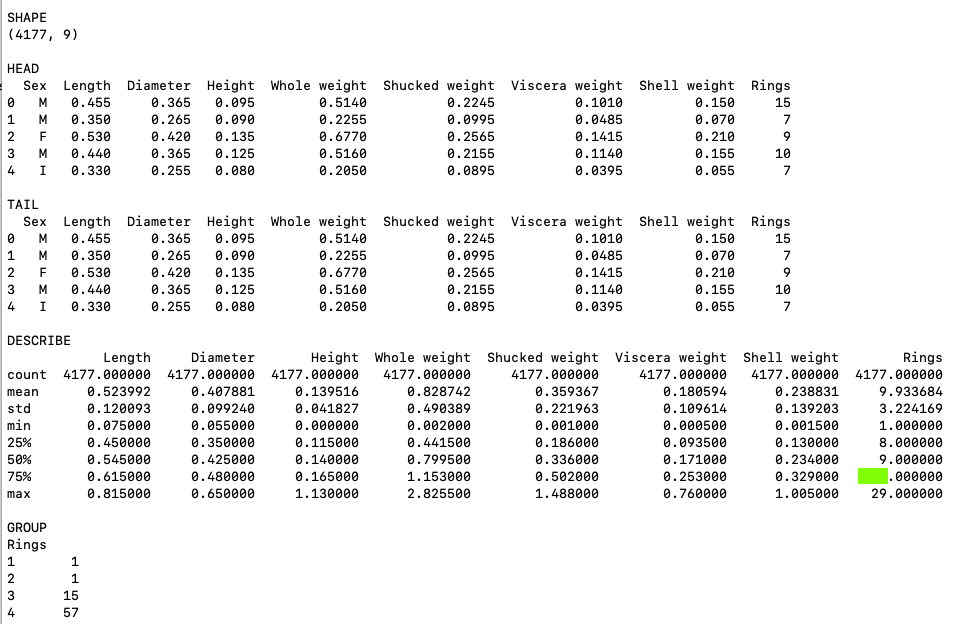# VP 400: Machine Learning (50 pts)

## Purpose

Develop very basic machine learning programs, following this tutorial:

## Install Libraries

```python3 -m pip install scipy python3 -m pip install numpy python3 -m pip install matplotlib python3 -m pip install pandas python3 -m pip install sklearn ```

We'll use the standard Iris flower data set.

The data set contains 150 records of measurements of iris flowers.

Create a program named ml1.py containing this code:

```from pandas import read_csv # Load dataset url = "https://raw.githubusercontent.com/jbrownlee/Datasets/master/iris.csv" names = ['sepal-length', 'sepal-width', 'petal-length', 'petal-width', 'class'] dataset = read_csv(url, names=names) # shape print(dataset.shape) # head print(dataset.head(20)) # descriptions print(dataset.describe()) # class distribution print(dataset.groupby('class').size()) ```
After a few seconds, it downloads the data and prints out a summary, as shown below.

There are 150 records with 5 attributes. There are four measurements of each iris, sorted into three possible classes.

We want to write a program that will learn to identify the class of new irises from the other attributes.## Visualizing Data with Histograms

Create a program named ml2.py containing this code:
```# Load libraries from pandas import read_csv from pandas.plotting import scatter_matrix from matplotlib import pyplot from sklearn.model_selection import train_test_split from sklearn.model_selection import cross_val_score from sklearn.model_selection import StratifiedKFold from sklearn.metrics import classification_report from sklearn.metrics import confusion_matrix from sklearn.metrics import accuracy_score from sklearn.linear_model import LogisticRegression from sklearn.tree import DecisionTreeClassifier from sklearn.neighbors import KNeighborsClassifier from sklearn.discriminant_analysis import LinearDiscriminantAnalysis from sklearn.naive_bayes import GaussianNB from sklearn.svm import SVC # Load dataset url = "https://raw.githubusercontent.com/jbrownlee/Datasets/master/iris.csv" names = ['sepal-length', 'sepal-width', 'petal-length', 'petal-width', 'class'] dataset = read_csv(url, names=names) # histograms dataset.hist() pyplot.show() ```
Histograms of the data appear, as shown below.

The attributes "petal-length" and "petal-width" have two clear groups, suggesting that they may be important attributes to determine the class.## Multivariate Plots

Create a program named ml3.py containing this code:
```# Load libraries from pandas import read_csv from pandas.plotting import scatter_matrix from matplotlib import pyplot from sklearn.model_selection import train_test_split from sklearn.model_selection import cross_val_score from sklearn.model_selection import StratifiedKFold from sklearn.metrics import classification_report from sklearn.metrics import confusion_matrix from sklearn.metrics import accuracy_score from sklearn.linear_model import LogisticRegression from sklearn.tree import DecisionTreeClassifier from sklearn.neighbors import KNeighborsClassifier from sklearn.discriminant_analysis import LinearDiscriminantAnalysis from sklearn.naive_bayes import GaussianNB from sklearn.svm import SVC # Load dataset url = "https://raw.githubusercontent.com/jbrownlee/Datasets/master/iris.csv" names = ['sepal-length', 'sepal-width', 'petal-length', 'petal-width', 'class'] dataset = read_csv(url, names=names) # scatter plot matrix scatter_matrix(dataset) pyplot.show() ```
A grid of charts appear, as shown below.

These charts show relationships between the attributes.

For example, look at the two charts outlined in red in the image below. The dots lie close to a line, with little scatter.

This means there is a strong correlation between "petal-length" and "petal-width". That they both contain the same information--measuring one of them is enough to predict the other.## Splitting the Data

We'll use 80% of the data to train the model, and the other 20% to test it. Create a program named ml4.py containing this code:
```# Load libraries from pandas import read_csv from pandas.plotting import scatter_matrix from matplotlib import pyplot from sklearn.model_selection import train_test_split from sklearn.model_selection import cross_val_score from sklearn.model_selection import StratifiedKFold from sklearn.metrics import classification_report from sklearn.metrics import confusion_matrix from sklearn.metrics import accuracy_score from sklearn.linear_model import LogisticRegression from sklearn.tree import DecisionTreeClassifier from sklearn.neighbors import KNeighborsClassifier from sklearn.discriminant_analysis import LinearDiscriminantAnalysis from sklearn.naive_bayes import GaussianNB from sklearn.svm import SVC # Load dataset url = "https://raw.githubusercontent.com/jbrownlee/Datasets/master/iris.csv" names = ['sepal-length', 'sepal-width', 'petal-length', 'petal-width', 'class'] dataset = read_csv(url, names=names) # Split-out validation dataset array = dataset.values X = array[:,0:4] y = array[:,4] X_train, X_validation, Y_train, Y_validation = train_test_split(X, y, test_size=0.20, random_state=1) # Spot Check Algorithms models = [] models.append(('LR', LogisticRegression(solver='liblinear', multi_class='ovr'))) models.append(('LDA', LinearDiscriminantAnalysis())) models.append(('KNN', KNeighborsClassifier())) models.append(('CART', DecisionTreeClassifier())) models.append(('NB', GaussianNB())) models.append(('SVM', SVC(gamma='auto'))) # evaluate each model in turn results = [] names = [] for name, model in models: kfold = StratifiedKFold(n_splits=10, random_state=1, shuffle=True) cv_results = cross_val_score(model, X_train, Y_train, cv=kfold, scoring='accuracy') results.append(cv_results) names.append(name) print('%s: %f (%f)' % (name, cv_results.mean(), cv_results.std())) ```
The accuracy of the models appears, as shown below. The number shown is the percent correct followed by its standard deviation.

Your numbers will differ somewhat because the learning process contains some randomness.

The Support Vector Machines (SVM) model had the highest accuracy when I did it.# Flag VP 400.1: Load Data (10 pts)

COVID19c.csv
(I made it from the sources listed at the bottom of this page. It is not perfect: I only identified 50 state governors, but there were 56 states in the raw case data. Please just regard it as random numbers for this project, not scientific evidence of anything.)

Load the data and print summaries, as shown below.

The flag is covered by a green rectangle in the image below.# Flag VP 400.2: Best Model (10 pts)

Test the same six models on the data you downloaded in the previous challenge.

Find the best model.

The flag is the name of that model, covered by a green rectangle in the image below.# Flag VP 400.3: Load Data (10 pts)

Abalone.csv
(I got it here.)

Load the data and print summaries, as shown below.

The flag is covered by a green rectangle in the image below.# Flag VP 400.4: Histogram (5 pts)

Continue using the abalone data.

What attribute has the histogram shown below?

The flag is the name of the attribute, covered by a green rectangle in the image below.# Flag VP 400.5: Multivariate Plots (5 pts)

Continue using the abalone data.

Find the multivariate plot matching the image shown below.

The flag is the name of the attribute covered by a green rectangle in the image below.# Flag VP 400.6: Best Model (10 pts)

Continue using the abalone data.

Remove the "Sex" column from the data.

Test the same six models on the data you downloaded in the previous challenge, using the other seven columns to predict "Rings".

Find the worst model. There are warnings that "The least populated class in y has only 1 members", as shown below, but just ignore them.

The flag is the name of that model, covered by a green rectangle in the image below.## References

Your First Machine Learning Project in Python Step-By-Step
Partisan composition of governors
COVID-19 Research and Information Resources
Coronavirus (Covid-19) Data in the United States

Posted 7-2-2020
Plaintext for 301.1 fixed 7-6-2020